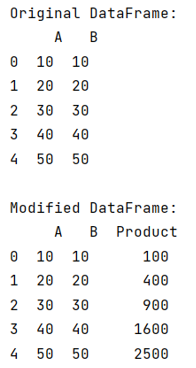# Multiply two columns in a pandas dataframe and add the result into a new column

Given a DataFrame, we need to multiply two columns in this DataFrame and add the result into a new column.
Submitted by Pranit Sharma, on July 23, 2022

Pandas is a special tool that allows us to perform complex manipulations of data effectively and efficiently. Inside pandas, we mostly deal with a dataset in the form of DataFrame. DataFrames are 2-dimensional data structures in pandas. DataFrames consist of rows, columns, and data.

To learn how to multiply two columns and add the result into a new column, we will first create a DataFrame, the dataframe will contain 2 columns initially and we will perform a row-wise product of both columns and store all the values in a list. Finally, we will assign this list to a new column of the DataFrame.

Let us understand with the help of an example,

## Python code to multiply two columns in a pandas dataframe and add the result into a new column

```# Importing pandas package
import pandas as pd

# Creating a Dictionary
d = {
'A':[10,20,30,40,50],
'B': [10,20,30,40,50]
}

# Creating a DataFrame
df = pd.DataFrame(d)

# Display original DataFrame
print("Original DataFrame:\n",df,"\n")

# Creating a list of values
list = []
for i in range(len(df['A'])):
a = (df['A'][i]*df['B'][i])
list.append(a)

# Creating a new column and assigning
# its values as the list defined above
df['Product'] = list

# Display modified DataFrame
print("Modified DataFrame:\n",df)
```

Output:What's New

Top Interview Coding Problems/Challenges!

Languages: » C » C++ » C++ STL » Java » Data Structure » C#.Net » Android » Kotlin » SQL
Web Technologies: » PHP » Python » JavaScript » CSS » Ajax » Node.js » Web programming/HTML
Solved programs: » C » C++ » DS » Java » C#
Aptitude que. & ans.: » C » C++ » Java » DBMS
Interview que. & ans.: » C » Embedded C » Java » SEO » HR
CS Subjects: » CS Basics » O.S. » Networks » DBMS » Embedded Systems » Cloud Computing
» Machine learning » CS Organizations » Linux » DOS
More: » Articles » Puzzles » News/Updates